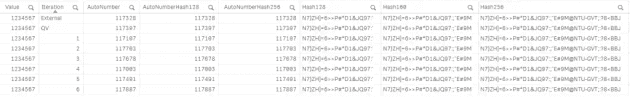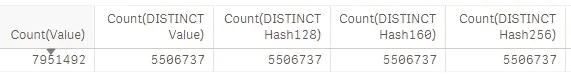# Comparing Autonumber, Autonumberhash128, Autonumberhash256, Hash128, Hash160 and Hash256 outputs in Qlik Sense and QlikView

October 14, 2018

There's often a discussion about what each of these autonumber/hash functions does in Qlik. We commonly see these functions used for creating integer key fields, anonymising data (for example, names in demo apps), and maintaining long string fields for later comparison (as the hashes are shorter than the strings they replace).Sample outputs from the random number generator, with all the functions present

To do this, I'm using the script below. I'm also keen to show outputs from QlikView vs Qlik Sense, and results of running the same script on another machine.

My observations are the following:
- AutoNumber/AutoNumberHash128/256 - different output per load as the value is typically based on the load order of the source data
- Hash128/160/256 - the same output, across every load. Stays the same between Qlik Sense and QlikView, and also between different machines

# The Process

I've opted to create random data, with an embedded control value. I'm doing this because I don't want to have the same distinct values in every sample, and I certainly don't want them in the same order (as I'll likely get the same integer outputs for the autonumber fields).

The process was:
- Generate a set of QVD files containing 1,000,000 random numbers, plus 1 control number (this is the value '123456'). These are sorted in ascending order, which means the control number should be at a different position in each QVD.
- Load all of these QVDs into an analysis app and compare the values for each function.

# The QVD generator

In the QVD generator, we use a simple load script to build the random numbers and autonumber/hash them.

``````// Load a control value into a temp table
// This will be the value we check the value of from each source
Data:
1234567						AS Random
AutoGenerate 1;

// Now concatenate 1,000,000 random values to the control value
// It's likely we will get some duplicates due to the limited range of values possible
Concatenate(Data)
Floor(rand()*10000000)		AS Random
AutoGenerate 1000000;

// Now sort this data to ensure the control value isn't the first record
// This is important for the autonumber functions as they tend to use load order
Sorted:
Random		                As Value
RESIDENT Data
ORDER BY Random;

// Now drop the data table
DROP TABLE Data;

// Calculate all of our hash and autonumber values
// The iteration field is used instead of stripping the filename
Hash:
Value,
Autonumber(Value)			AS AutoNumber,
Autonumberhash128(Value)		AS AutoNumberHash128,
Autonumberhash256(Value)		AS AutoNumberHash256,
Hash128(Value)			AS Hash128,
Hash160(Value)			AS Hash160,
Hash256(Value)			AS Hash256,
6 as Iteration
RESIDENT Sorted;

// Store and drop the hash table
STORE Hash INTO [lib://StackExchange/Rand-6.qvd] (qvd);
DROP TABLE Hash;``````

Doing this, I produced 7 files - 6 from Qlik Sense (September 2018) and 1 from running the same script in Qlik View (12.10 SR3).Random Number QVDs

These were then loaded straight into a Qlik Sense app, along with an excel file containing similar results generated through Qlik Cloud.

# The analysis

After loading these results into an app, we can see that only the hash remains consistent for our test value. This remains true:
- Between different Qlik Sense sessions
- Between different Qlik Sense servers (desktop, enterprise and Qlik Cloud)
- Between QlikView and Qlik SenseResults for the control value, 1234567

All 8 iterations resulted in the same hash values, while the autonumber values were predictably variable - their order is driven mainly by load order. If the values and load order of the source hadn't changed then it is possible that the autonumber values would have been the same between tests.

# Using a "sample load" to do this on real data

The other way I could have done this is using real data, and the Qlik "Sample Load". This loads a random sample from a source table, and I'd only need to inject a control value, then sort it by all values as I did above.

# A last thought - collisions

A challenge when using large data sets and hash fields is the chance of collisions and what this might mean for any analysis relying on this field.

As hash outputs have a fixed length (in Qlik this is 22, 27 and 43 characters, for 128, 160 and 256 bit hashes respectively) and the input can be of any length, there is a chance that the same hash is produced by different inputs.On our small data set, the distinct hash count was as expected (over 7.9m values)

This is, however, very unlikely to present a problem on Qlik-sized data sets (e.g. up to 1 billion unique values, which is the software limit).

From Dave, who writes to learn things. Thoughts and views are his own.